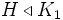# Upper join-closed subgroup property

This article defines a subgroup metaproperty: a property that can be evaluated to true/false for any subgroup property
View a complete list of subgroup metaproperties
View subgroup properties satisfying this metaproperty| View subgroup properties dissatisfying this metaproperty
VIEW RELATED: subgroup metaproperty satisfactions| subgroup metaproperty dissatisfactions

## Definition

### Definition with symbols

A subgroup property$p$ is said to be upper join-closed if given$H \le G$ and$K_i, i \in I$ are intermediate subgroups of$G$ containing$H$ (indexed by a nonempty set$I$) and$H$ satisfies$p$ in each$K_i$, we have that$H$ satisfies$p$ in the join of subgroups$\langle K_i \rangle_{i \in I}$.

## Related notions

Given a subgroup property$p$ that is identity-true, upper join-closed and also satisfies the intermediate subgroup condition, we can, given any subgroup$H$ of$G$ associate a unique largest subgroup$M$ containing$H$ for which$H$ satisfies$p$ in$M$.

Such a subgroup property is termed an izable subgroup property and the$M$ that we get is termed the izing subgroup of$H$ for that subgroup property.

## Properties satisfying it

### Normality

Normality is an upper join-closed subgroup property, viz, if$H \le G$ and$K_1, K_2$ are intermediate subgroups such that$H \triangleleft K_1$ and$H \triangleleft K_2$, then$H \triangleleft $.

### Central factor

The property of being a central factor is also upper join-closed, in fact, it is izable.